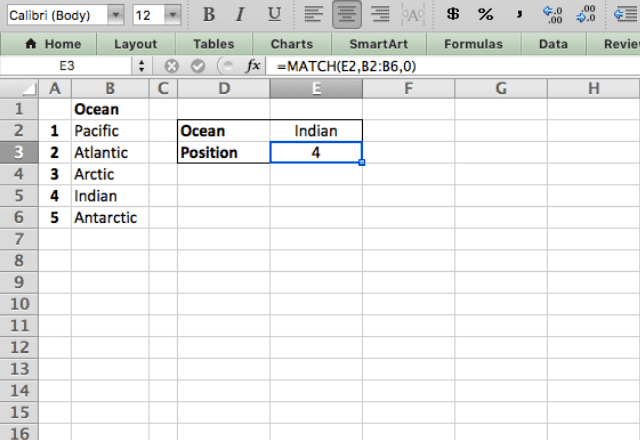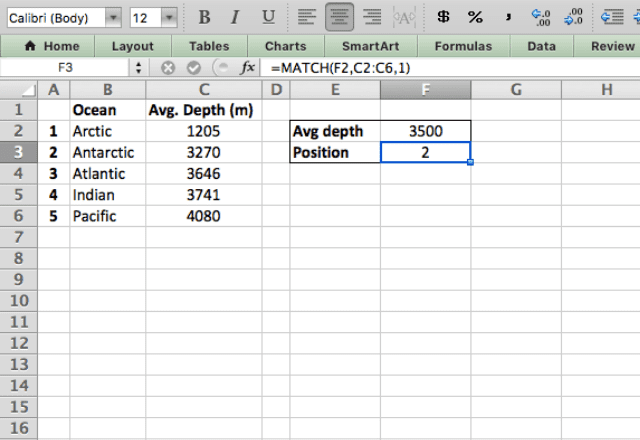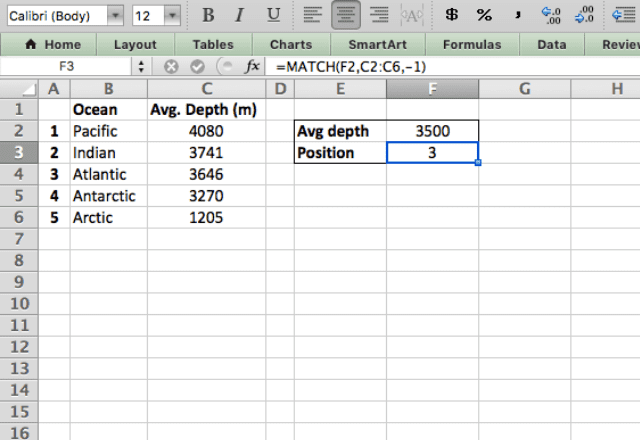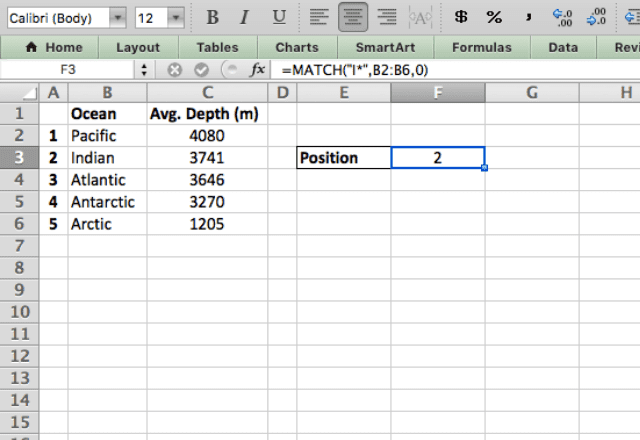# How to use the MATCH Excel functionThe MATCH Excel function tells you the position of a value within a range of cells. The function on its own can be useful in certain situations, and it can be combined with other functions for even more utility (check out the INDEX MATCH Excel combination).

### Syntax

=MATCH(lookup_value, lookup_array, [match_type])
• lookup_value is the value you’re searching for
• lookup_array is the array that you want to search
• match_type is the type of match that you want (see below for details). If left blank, it will default to type 1 which is an approximate match
##### Match type

The match_type parameter has three possible values: 0, 1, and -1.

• 0 specifies an exact match
• 1 specifies an approximate match, where the value found will be equal to or less than the lookup_value. Values must be sorted in ascending order. Use an approximate match when your data may not have the exact value you’re looking for
• -1 specifies an approximate match, where the value found will be equal to or greater than the lookup_value. Values must be sorted in descending order

When using approximate matches (match_type 1 or -1) the data must be sorted in ascending (1) or descending (-1) order. If the data isn’t sorted, you’ll probably get an incorrect answer!

### How to use the MATCH Excel function

#### Exact match

I’ll start with a basic example using the MATCH function to find an exact match (with match_type 0). I have a list of oceans and I want to know where in the list the Indian Ocean is situated.

The formula here is =MATCH(E2,B2:B6,0) and the function correctly tells me that the Indian Ocean is 4th in the list.#### Approximate match (ascending order)

Now I’ll use match_type 1 to give me an approximate match. This is useful when you know you don’t have an exact match, especially when you’re dealing with numerical data.

In this example I want to get the position of the ocean with average depth closest to 3500m.The formula here is =MATCH(F2,C2:C6,1). As mentioned before, a match_type of 1 gives an approximate match where the result is equal to or less than the lookup_value. That’s why it gave me the position in the list of the Antarctic Ocean, even though the Atlantic is closer to 3500m average depth.

Also remember that for this type of match, the data must be sorted in ascending order!

#### Approximate match (descending order)

The other type of approximate match is match_type -1 which gives the closest value equal to or greater than the lookup_value.

In this case the data must be sorted in descending order.The formula here is =MATCH(F2,C2:C6,-1). Now you can see the difference between the two match_types – in both cases I asked for the ocean closest to 3500m depth, but got two different answers!

This is something to keep in mind when choosing which type of match you want.

#### MATCH with wildcards

Wildcards (characters * and ?) can be used to represent any value. The asterisk (*) represents any number of any character, while the question mark (?) represents one of any character. The MATCH Excel function can use these wildcards when searching for an exact match.

Pretend I wanted to find the position of the Indian Ocean in my list, but I forget how to spell it – all I know is that it starts with an “I”. I can use the * wildcard to represent the other characters.

So by typing =MATCH(“I*”,B2:B6,0) the formula correctly gives me the position of the Indian Ocean!You’ve now seen a number of different uses of the MATCH function. Remember, it provides the position of a value in an array.

If you want to get more use out of the MATCH function, consider combining it with the INDEX function, which can help make it even more useful by returning a value instead of just the position.

### Tips when using MATCH Excel

• When the match_type parameter is left blank, the function defaults to an approximate match (type 1)
• Always remember to sort the data when using an approximate match. Type 1 needs data sorted in ascending order, and Type -1 in descending order
• The MATCH function is not case sensitive
• If you have duplicates in your data, the function will return the first match
• Check out the INDEX MATCH function combination to get more out of the MATCH function